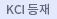#다국어 입력

http://chineseinput.net/에서 pinyin(병음)방식으로 중국어를 변환할 수 있습니다.

변환된 중국어를 복사하여 사용하시면 됩니다.

예시)
• 中文 을 입력하시려면 zhongwen을 입력하시고 space를누르시면됩니다.
• 北京 을 입력하시려면 beijing을 입력하시고 space를 누르시면 됩니다.
닫기

검색결과 좁혀 보기

선택해제
• 좁혀본 항목 보기순서

• 원문유무
• 음성지원유무
• 원문제공처
• 등재정보
• 학술지명
• 주제분류
• 발행연도
• 작성언어
• 저자

오늘 본 자료

• 오늘 본 자료가 없습니다.
더보기
• 무료
• 기관 내 무료
• 유료
•섭동 추정 프로세스를 이용한 불확실 시스템에 대한 강인 칼만 필터링 기법

A robust Kalman filtering method for uncertain stochastic systems is suggested by adopting a perturbation estimation process which is to reconstruct total uncertainty with respect to the nominal state transition equation. The predictor and corrector of discrete Kalman filter are reformulated with the perturbation estimator. Successively, the state and perturbation estimation error dynamics and the corresponding error covariance propagation equations are derived as well. Finally we have the recursive algorithm of Combined Kalman Filter-Perturbation Estimator (CKF). The proposed combined Kalman filter-perturbation estimator has the property of integrating innovations and the adaptation capability to system uncertainties. A numerical example is shown to demonstrate the effectiveness of the proposed scheme.

•평판 디스플레이 비전 정렬 시스템의 기구학 및 제어

The kinematics and control problem of a visual alignment system is investigated, which plays a crucial role in the fabrication process of flat panel displays. The first solution is the inverse kinematics of a 4PPR parallel alignment mechanism. It determines the driving distance of each joint to compensate the misalignment between mask and panel. Second, an efficient vision algorithm for fast alignment mark recognition is suggested, where by extracting essential feature points to represent the geometry of a mark, the geometric template matching enables much faster object recognition comparing with the general template matching. Finally, the overall visual alignment process including the kinematic solution, vision algorithm, and joint control is implemented and experimental results are given.

•이족보행 로봇의 무게중심 실시간 추정에 관한 연구

In this paper, a closed-loop observer to extract the center of mass (CoM) of a bipedal robot is suggested. Comparing with the simple conversion method of just using joint angle measurements, it enables to get more reliable estimates by fusing both joint angle measurements and F/T sensor outputs at ankle joints. First, a nonlinear-type observer is constructed to estimate the flexible rotational motion of the biped in the extended Kalman filter framework. It adopts the flexible inverted pendulum model which is appropriate to address the flexible motion of bipeds, specifically in the single support phase. The predicted estimates of CoM in terms of the flexible motion observer are combined with measurements (that is, output of the CoM conversion equation with joint angles). Then, we have final CoM estimates depending on the weighting values which penalize the flexible motion model and the CoM conversion equation. Simulation results show the effectiveness of the proposed algorithm.

••3차원 하이브리드 비전 정렬 시스템에 관한 연구

In this paper, suggested is a hybrid-type visual alignment system to align mask and panel in 3-D space, where mask and panel are to be controlled independently by two individual positioning mechanisms in order to compensate for spatial misalignments. In the hybrid visual alignment system, the below 4-PPR parallel mechanism provides in-plain motions to pattern mask like the other conventional alignment systems while the above 4-RPS parallel mechanism is to move glass panel to achieve a complete spatial alignment. For the control of the hybrid alignment system, first, inverse kinematic solutions for the parallel mechanisms are given to determine the driving distance of each active joint, and also an efficient way to determine the spatial alignment error is developed by exploiting three in-plane cameras.

해외이동버튼